Home

# The Infinite limit of random permutations avoiding patterns of length three

## Abstract

For$$\tau \in {S_3}$$ , let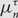$$\mu _n^\tau$$ denote the uniformly random probability measure on the set of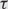$$\tau$$ -avoiding permutations in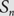$${S_n}$$ . Let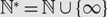$${\mathbb {N}^*} = {\mathbb {N}} \cup \{ \infty \}$$ with an appropriate metric and denote by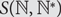$$S({\mathbb{N}},{\mathbb{N}^*})$$ the compact metric space consisting of functions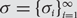$$\sigma {\rm{ = }}\{ {\sigma _i}\} _{i = 1}^\infty {\rm{ }}$$ from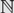$$\mathbb {N}$$ to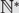$${\mathbb {N}^ * }$$ which are injections when restricted to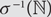$${\sigma ^{ - 1}}(\mathbb {N})$$ ; that is, if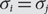$${\sigma _i}{\rm{ = }}{\sigma _j}$$ ,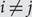$$i \ne j$$ , then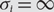$${\sigma _i} = \infty$$ . Extending permutations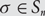$$\sigma \in {S_n}$$ by defining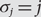$${\sigma _j} = j$$ , for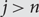$$j \gt n$$ , we have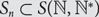$${S_n} \subset S({\mathbb{N}},{{\mathbb{N}}^*})$$ . For each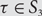$$\tau \in {S_3}$$ , we study the limiting behaviour of the measures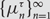$$\{ \mu _n^\tau \} _{n = 1}^\infty$$ on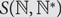$$S({\mathbb{N}},{\mathbb{N}^*})$$ . We obtain partial results for the permutation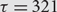$$\tau = 321$$ and complete results for the other five permutations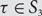$$\tau \in {S_3}$$ .

Hide All

# The Infinite limit of random permutations avoiding patterns of length three

## Metrics

### Full text viewsFull text views reflects the number of PDF downloads, PDFs sent to Google Drive, Dropbox and Kindle and HTML full text views.

Total number of HTML views: 0
Total number of PDF views: 0 *Loading metrics...

### Abstract viewsAbstract views reflect the number of visits to the article landing page.

Total abstract views: 0 *Loading metrics...

* Views captured on Cambridge Core between <date>. This data will be updated every 24 hours.

Usage data cannot currently be displayed